Mathematics Letters
Volume 1, Issue 3, October 2015, Pages: 17-19

A Partial Answer to Sidorenko’s Conjecture on a Correlation Inequality for Bipartite Graphs

Danyal Soybas, Onur Alp Ilhan

Department of Mathematics, Faculty of Education, Erciyes University, Kayseri, Turkey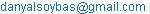(D. Soybas)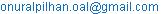(O. A. Ilhan)

Danyal Soybas, Onur Alp Ilhan. A Partial Answer to Sidorenko’s Conjecture on a Correlation Inequality for Bipartite Graphs.Mathematics Letters.Vol.1, No. 3, 2015, pp. 17-19. doi: 10.11648/j.ml.20150103.11

Abstract: Sidorenko conjectured an integral inequality for a product of functions h(xi, yi) where the diagram of the product is a bipartite graph G in . We answered the conjecture positively when the function h is multiplicative or additive separable with respect to variables x and y.

Keywords: Sidorenko’s Conjecture, Bipartite Graph, Lebesgue Measure, Measurable Function

Contents

1. Introduction

Denote the Lebesgue measure on [0, 1] by µ. Let a fuction h(x, y) be bounded, nonnegative and measurable on [0, 1]2. Let G be a bipartite graph where vertices u1, u2,…,un form the first part and v1, v1,…, vm the second part. We denote by E the set of pairs (i, j) for which ui and vj are adjacent in G. So |E| is the number of edges. The following conjecture was discussed in works [2,3] published in Russian. The same arguments were explained in detail again in .

Conjecture 1 . For any bipartite graph G and any function h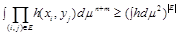(1)

Two particular cases of Inequality 1 (G is a path, h is symmetric; G is a path of length 3, h is not necessarily symmetric) were proven in [4,5] and , respectively.

One might conjecture that the considered products of functions are always non-negative correlated. Unfortunately, this is not the case. For instance, it would require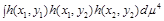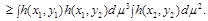However, a counterexample to the last inequality was found in .

Conjecture 2 . Let the numbers of edges and vertices of a bipartite graph G satisfy the conditions |E| ≥ n; |E| ≥ m. Then, for any functions h, f1, f2,…, fn, g1, g2,…, gm,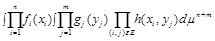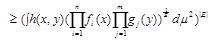(2)

Inequality 2 is essentially stronger than inequality 1. For instance, inequality 2 implies :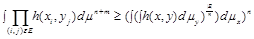(3)

Conjecture 1 and 2 were proven in  for various classes of graphs.

2. Main Results

We start by mentioning a simple example as follows:

Let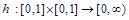be a function given by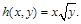h is trivially non-negative, bounded and Lebesgue measurable. Let G be a finite simple bipartite graph with {u1, u2} such that u1 to both v1 and v2 and u2 to both v1 and v2. Siderenko’s conjecture is positively confirmed because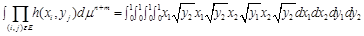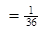Lemma 3 . Suppose that 1 ≤ r < ∞ and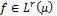is non-negative. Then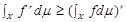. If r > 1, then equality holds if and only if f is (essentially) constant.

In order to make the understanding of Theorem 4 easier, we give a simpler format of it as an example. First let G be a simple bipartite graph from u1 to both v1 and v2 and the function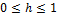be measurable on [0, 1]2. Let h be written as h(x,y)=h(x).g(y)

I1=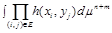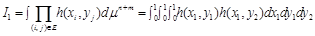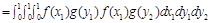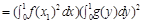By Lemma 3, we get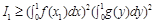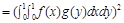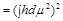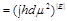Now we can give Theorem 4 which is a generalization of above mentioned argument.

Theorem 4. For any bipartite graph G and any non-negative, bounded and Lebesgue measurable function h such that h(x,y)=f(x).g(y) we have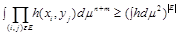Proof: Let G be a finite simple bipartite graph with bipartition {u1,u2,,…um}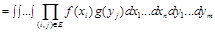where d(ui) is the degree of ui.

By Lemma 3 we know that the inequality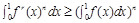satisfies for all natural number n.

By using Fubini’s Theorem we have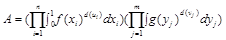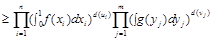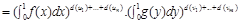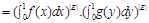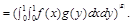Hence the proof was completed.

Now under the same assumption of G given before Theorem 4, let the functionbe written as h(x,y)=f(x)+g(y) which is measurable on [0, 1]2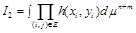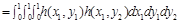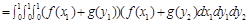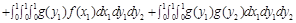Since the integrands at second and third order in above expression are the same, by Lemma 3 we have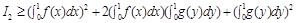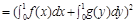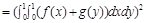Theorem 5. For any bipartite graph G and any function h such that h(x,y)=h(x)+g(y), we haveProof: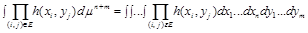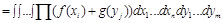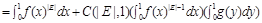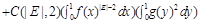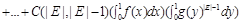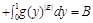By Lemma 3 we get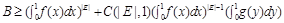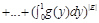By Binom expansion we get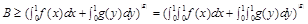which completed the proof.

Following Theorem 6 which was given in  can be considered as an useful means to investigate whether our Theorem 4 and Theorem 5 can be generalized to any bounded, non-negative and Lebesgue measurable function h.

Theorem 6 . Suppose than n is a positive integer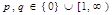, not both of p, q are zero,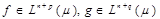, and f and g non-negative. Then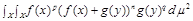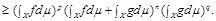If neither f nor g is the zero function then if n + p > 1, equality implies that f is constant, and if n + q >1, equality implies that g is constant.

Remark: We do not know whether Theorem 4 is valid for all non-negative, bounded and Lebesgue measurable functions h. If we can show that Theorem 4 is true for any function h such that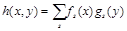where fs(x) and gs(y) are polynomials. Then by using Theorem 4, Theorem 5 and Theorem 6, we can easily generalize it to all h by using well-known Weierstrass approximation theorem, because polynomials are uniformly dense in C([0, 1]).

Acknowledgements

We would like to thank to Prof. Dr. Peter Johnson for his valuable suggestions.

References

1. Johnson P., Soybaş D., An Integral Inequality For Probability Spaces, International Journal of Mathematics and Computer Science, vol.8, pp.1-3, 2013.
2. Sidorenko,A.F, Inequalities for functional generated by bipartite graphs (in Russian), Discrete Mathematics and Applications 3(3) 50-65(1991).
3. Sidorenko,A.F., Exremal problems in graph theory and inequalities in functional analysis (in Russian). In Lupanov,O.B, ed.,Proceedings of the Soviet Seminar on Discrete Mathematics and it Applications (in Russian), pp. 99-105. Moscow: Moscow State University 1986, MR88m:05053.
4. Mulholland,H.P.,Smith,C.A.B., An inequality arising in genetical theory, Amer. Math. Mon. 66,673-683 (1959).
5. Blakley, G.R, Roy,P., Hölder type inequality for symmetrical matrices with nonnegative entries. Proc.Am.Math.Soc. 16,1244-1245 (1965).
6. Atkinson,F.V., Watterson,G.A.,Moran, P.A.D., A matrix inequality. Q.J.Math. Oxf. II Ser. 11, 137-140 (1960).
7. London, D., Two equalities in nonnegative symmetric matrices. Pac. J. Math.16, 515-536(1966).
8. Sidorenko, A., A Correlation Inequality for Bipartite Graphs., Graphs and Combinatorics (1993) 9, 201-204.

 Contents 1. 2.
Article ToolsAbstractPDF(177K)